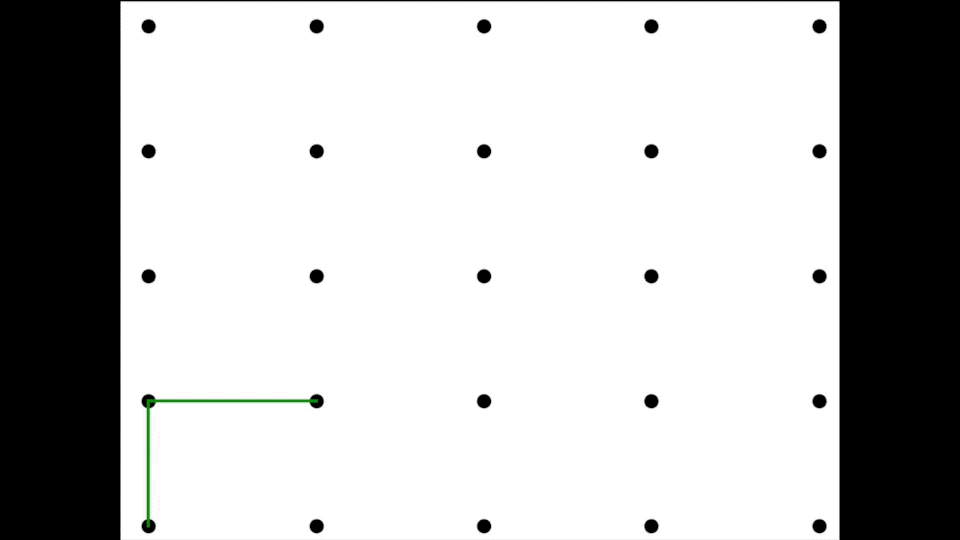# Random Walkers walking on a 2D grid

### Problem

Alice (starts from lower left corner) will walk north(up) or east(right) with a probability of 50% each. At the same time and at the same speed, Bob (starts form upper right corner) will walk south (down) and west (left) with equal probabilities. What is the probability that they will meet?

### Alice Random Walk

The following code simulates the random walk of Alice:

```# Author: Manas Sharma
# Random walker (Alice) walking on a 2d 4x4 grid.
import matplotlib.pyplot as plt
import numpy as np
# import matplotlib.animation as animation

# Points to draw the grid
x=[1,1,1,1,1,2,2,2,2,2,3,3,3,3,3,4,4,4,4,4,5,5,5,5,5]
y=[1,2,3,4,5,1,2,3,4,5,1,2,3,4,5,1,2,3,4,5,1,2,3,4,5]
# Draw the grid points
plt.scatter(x,y,c='black',s=50)
plt.title('Random walk of Alice')

# Starting position of Alice
pos = np.array([1,1])

# Arrays/Lists to store some positions to draw them later.
posX = []
posY = []
posX.append(pos)
posY.append(pos)

# Simulate the random walk upto nsteps steps
nsteps=8
for i in range(nsteps):
randno=np.random.random_integers(1,2)
if randno==1:# Right
if pos<5:
pos = pos + [1,0]
else:
pos = pos + [0,1]
else: # Up
if pos<5:
pos = pos + [0,1]
else:
pos = pos + [1,0]

posX.append(pos)
posY.append(pos)
# Draw the paths
plt.plot(posX,posY,c='green',linewidth=3)
# Pause for animation
plt.pause(0.5)

plt.show()
```

### Output### Bob Random Walk

The following code the random walk of Bob:

```# Author: Manas Sharma
# Random walker (Bob) walking on a 2d 4x4 grid.
import matplotlib.pyplot as plt
import numpy as np
# import matplotlib.animation as animation

# Points to draw the grid
x=[1,1,1,1,1,2,2,2,2,2,3,3,3,3,3,4,4,4,4,4,5,5,5,5,5]
y=[1,2,3,4,5,1,2,3,4,5,1,2,3,4,5,1,2,3,4,5,1,2,3,4,5]
# Draw the grid points
plt.scatter(x,y,c='black',s=50)
plt.title('Random walk of Bob')

# Starting position of Bob
pos = np.array([5,5])

# Arrays/Lists to store some positions to draw them later.
posX = []
posY = []
posX.append(pos)
posY.append(pos)

# Simulate the random walk upto nsteps steps
nsteps=8
for i in range(nsteps):
randno=np.random.random_integers(1,2)
if randno==1:# Left
if pos>1:
pos = pos - [1,0]
else:
pos = pos - [0,1]
else: # Down
if pos>1:
pos = pos - [0,1]
else:
pos = pos - [1,0]

posX.append(pos)
posY.append(pos)
# Draw the paths
plt.plot(posX,posY,c='orange',linewidth=3)
# Pause for animation
plt.pause(0.5)

plt.show()
```

### Alice and Bob Simultaneous Random Walks

The following code simulates the random walk of Alice and Bob starting simultaneously and going at the same speed.

```# Author: Manas Sharma
# Random walkers walking on a 2d 4x4 grid.

import matplotlib.pyplot as plt
import numpy as np
# import matplotlib.animation as animation

# Points to draw the grid
x=[1,1,1,1,1,2,2,2,2,2,3,3,3,3,3,4,4,4,4,4,5,5,5,5,5]
y=[1,2,3,4,5,1,2,3,4,5,1,2,3,4,5,1,2,3,4,5,1,2,3,4,5]
# Draw the grid points
plt.scatter(x,y,c='black',s=50)
plt.title('Random walk of Alice and Bob')

# Starting positions of Alice and Bob
posA = np.array([1,1])
posB = np.array([5,5])

# Arrays/Lists to store some positions to draw them later.
posX_A = []
posY_A = []
posX_B = []
posY_B = []
posX_A.append(posA)
posY_A.append(posA)
posX_B.append(posB)
posY_B.append(posB)

# Simulate the random walk upto insteps steps
nsteps=8
for i in range(nsteps):
randno=np.random.random_integers(1,2)
if randno==1:# Right
if posA<5:
posA = posA + [1,0]
else:
posA = posA + [0,1]
else: # Up
if posA<5:
posA = posA + [0,1]
else:
posA = posA + [1,0]

posX_A.append(posA)
posY_A.append(posA)

randno=np.random.random_integers(1,2)
if randno==1:# Left
if posB>1:
posB = posB - [1,0]
else:
posB = posB - [0,1]
else: # Down
if posB>1:
posB = posB - [0,1]
else:
posB = posB - [1,0]

posX_B.append(posB)
posY_B.append(posB)

# Draw the paths
plt.plot(posX_A,posY_A,c='green', linewidth=3)
plt.plot(posX_B,posY_B,c='orange', linewidth=3)
# Pause for animation
plt.pause(1.0)

plt.show()
```

### Probability calculation

The following code runs the complete simulation and calculates the probability of Alice and Bob meeting:

```# Author: Manas Sharma
# Probablitiy of Random walkers meeting on a 2d 4x4 grid.

import matplotlib.pyplot as plt
from matplotlib.pyplot import figure
import numpy as np
# import matplotlib.animation as animation

figure(figsize=(8, 8), dpi=120)

# Points to draw the grid
x=[1,1,1,1,1,2,2,2,2,2,3,3,3,3,3,4,4,4,4,4,5,5,5,5,5]
y=[1,2,3,4,5,1,2,3,4,5,1,2,3,4,5,1,2,3,4,5,1,2,3,4,5]

# Number of total events.
Ntrials = 500

# Counter to keep track of number of times they have met
nmeet = 0

# Run the different trials
for ntr in range(1,Ntrials+1):
# Starting positions of Alice and Bob
posA = np.array([1,1])
posB = np.array([5,5])
# Arrays/Lists to store some positions to draw them later.
posX_A = []
posY_A = []
posX_B = []
posY_B = []
posX_A.append(posA)
posY_A.append(posA)
posX_B.append(posB)
posY_B.append(posB)
# Simulate the random walk upto nsteps steps
nsteps=8
for i in range(nsteps):
#Plot the grid points
plt.scatter(x,y,c='black',s=50)
randno=np.random.random_integers(1,2)
if randno==1:# Right
if posA<5:
posA = posA + [1,0]
else:
posA = posA + [0,1]
else: # Up
if posA<5:
posA = posA + [0,1]
else:
posA = posA + [1,0]

posX_A.append(posA)
posY_A.append(posA)

randno=np.random.random_integers(1,2)
if randno==1:# Left
if posB>1:
posB = posB - [1,0]
else:
posB = posB - [0,1]
else: # Down
if posB>1:
posB = posB - [0,1]
else:
posB = posB - [1,0]

posX_B.append(posB)
posY_B.append(posB)

# Draw the paths
plt.plot(posX_A,posY_A,c='green',linewidth=3,alpha=0.4)
plt.plot(posX_B,posY_B,c='orange',linewidth=3,alpha=0.4)

# Highlight the current path
plt.plot([posX_A[-1],posX_A[-2]],[posY_A[-1],posY_A[-2]],c='green',linewidth=4)
plt.plot([posX_B[-1],posX_B[-2]],[posY_B[-1],posY_B[-2]],c='orange',linewidth=4)

# Check if they met?
if posB==posA:
if posA==posB:
# Increment meeting counter
nmeet = nmeet + 1
plt.scatter(posA,posA,c='red',s=250)
plt.title('Nmeet = '+str(nmeet)+';      Ntrials = '+str(ntr)+';      Probability = '+str(round(probability,4)))
plt.pause(1.0)
# Calculate probability
probability = nmeet/ntr
plt.title('Nmeet = '+str(nmeet)+';      Ntrials = '+str(ntr)+';      Probability = '+str(round(probability,4)))
#Pause for animation
plt.pause(0.1)
plt.clf()

# Clear previous plot

print(probability)

plt.show()
```

### REFERENCES

Inspiration:[wpedon id="7041" align="center"]